# Removable set

Jump to: navigation, searchof points of the complex planefor a certain classof functions analytic in a domainA compact set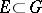such that any functionof classin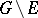can be continued as a function of classto the whole domain. The situation may be described in other words by saying that "the set E is set, removable for a class of functionsremovable for the class K" or that "E is a null-set for the class K" , briefly: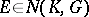. It is assumed that the complementis a domain and that the classis defined for any domain.

According to another definition, a setis removable for a class,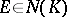, if the fact thatis a function of classin the complement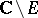implies that. Here the membership relationsandare generally speaking not equivalent.

A first result on removable sets was the classical Cauchy–Riemann theorem on removable singularities: If a functionis analytic and bounded in a punctured neighbourhoodof a point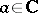, then it can be continued analytically to. A wider statement of the question (Painlevé's problem) is due to P. Painlevé: To find necessary and sufficient conditions on a setin order that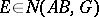, where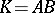is the class of all bounded analytic functions (cf. ). Painlevé himself found a sufficient condition:must have linear Hausdorff measure zero. Necessary and sufficient conditions for Painlevé's problem were obtained by L.V. Ahlfors (cf. ):if and only ifhas zero analytic capacity. There exists an example of a setof positive length but zero analytic capacity. On removable sets for different classes of analytic functions of one complex variable and related unsolved problems see , , , .

In the case of analytic functionsof several complex variables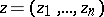,, the statement of the problem on removable sets is changed by virtue of the classical Osgood–Brown theorem: Ifis a regular analytic function in a domain, except possibly on a compact setfor which the complementis connected, thencan be continued analytically to the whole domain. For other theorems on removable sets for, as well as connections with the concept of a domain of holomorphy, see e.g. , .

The problem of removable sets can also be posed for harmonic, subharmonic and other functions. E.g., letbe a domain in Euclidean space,, letbe compact,, let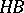be the class of bounded harmonic functions, and letbe the class of harmonic functions with finite Dirichlet integral. Then the membership relationsand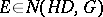are equivalent and are valid if and only if the capacity ofis zero (cf. , ).